# 雕刻时光 Sculpting in time

Every story has a beginning and an end.

## TF-MPI-Distributed-Training

Tensorflow MPI-based Distributed Training

## Horovod

I have written an article about how to build a distributed training cluster on Raspberry Pie 4 using the distributed training system that comes with TF2.0. However, there is a drawback: we need to start the training program at each node, and the distributed training will only work after all the nodes are started. MPI is mainly used in the field of supercomputing. Building MPI cluster on Raspberry, firstly, it can be used to learn distributed computing on supercomputing, and secondly, it can observe the performance of TF> distributed training on ARMv8 in practice.

## Review

The development of horovod can be started by researching this paper Horovod: fast and easy distributed deep learning in Tensorflow.

There are two common types of distributed parallel processing, one is data parallelism and the other is model parallelism, which was discussed in a previous article, and the advantage of data parallelism is that it is relatively simple.

Data parallel process. 1:

Distribute a copy of the model code on each node and run it.

• Read data set per node to start training
• Calculate the gradient of the model from the loss function
1. merge the calculated gradients at each node to calculate the new average gradient.

2. update the parameters and weights of the model at each node according to the new gradient calculated.

3. repeat the above process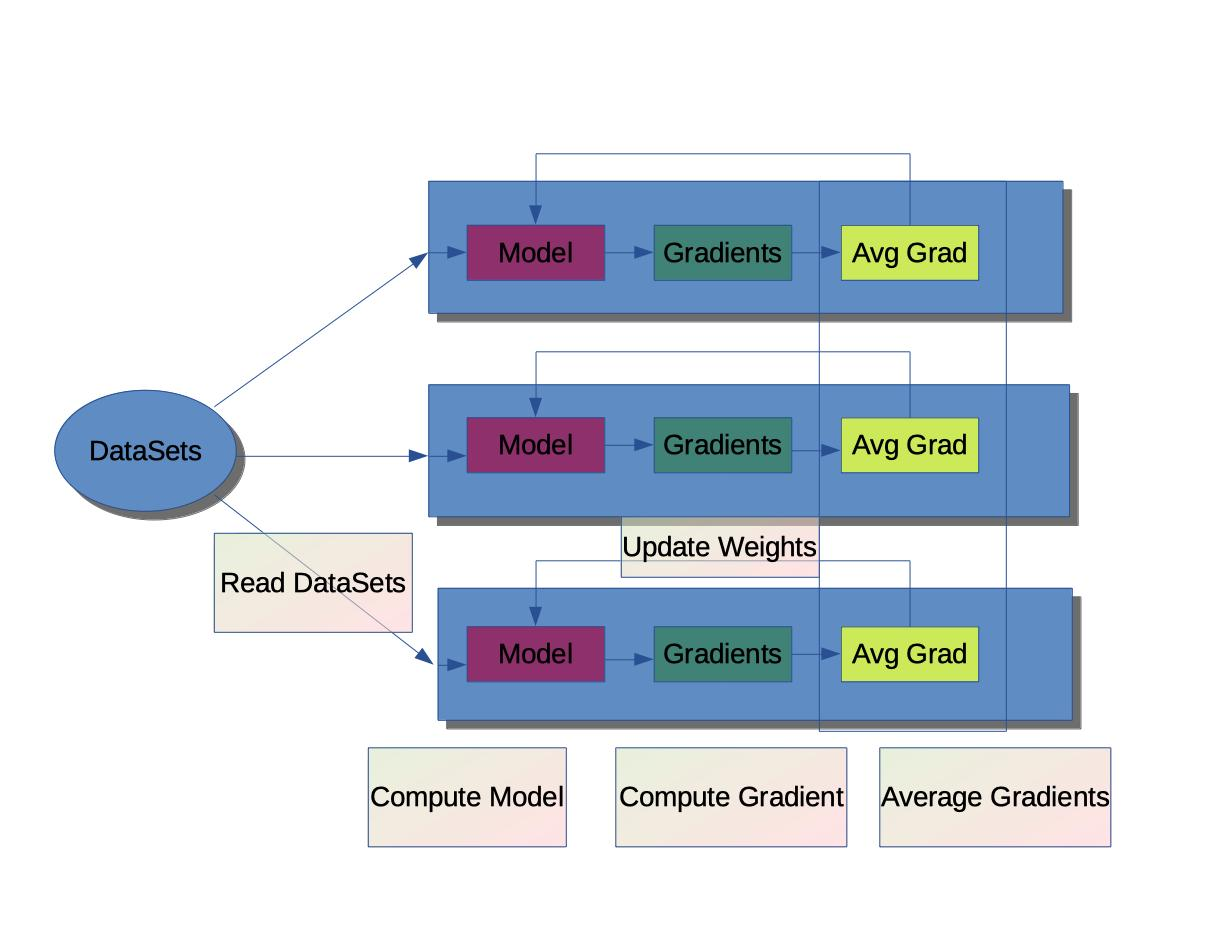The early TF distributed training for PS architecture, Worker training model, the gradient obtained is sent to parameter server, PS service will average the collected data, developers need to start Worker and PS on each node, then TF through the service discovery mechanism to access the whole training cluster, so there will be two bottlenecks.

1. when there is only one PS service, the PS load will be too high.
1. When there are multiple PS and multiple workers, the bottleneck for training occurs at the network bandwidth level.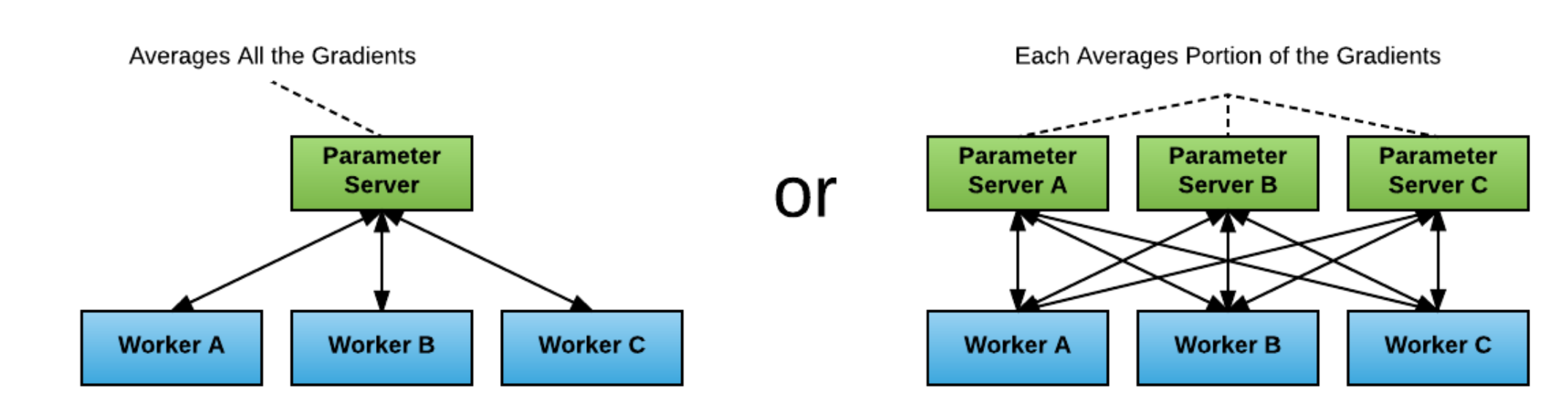Next comes the Ring-All-Reduce algorithm: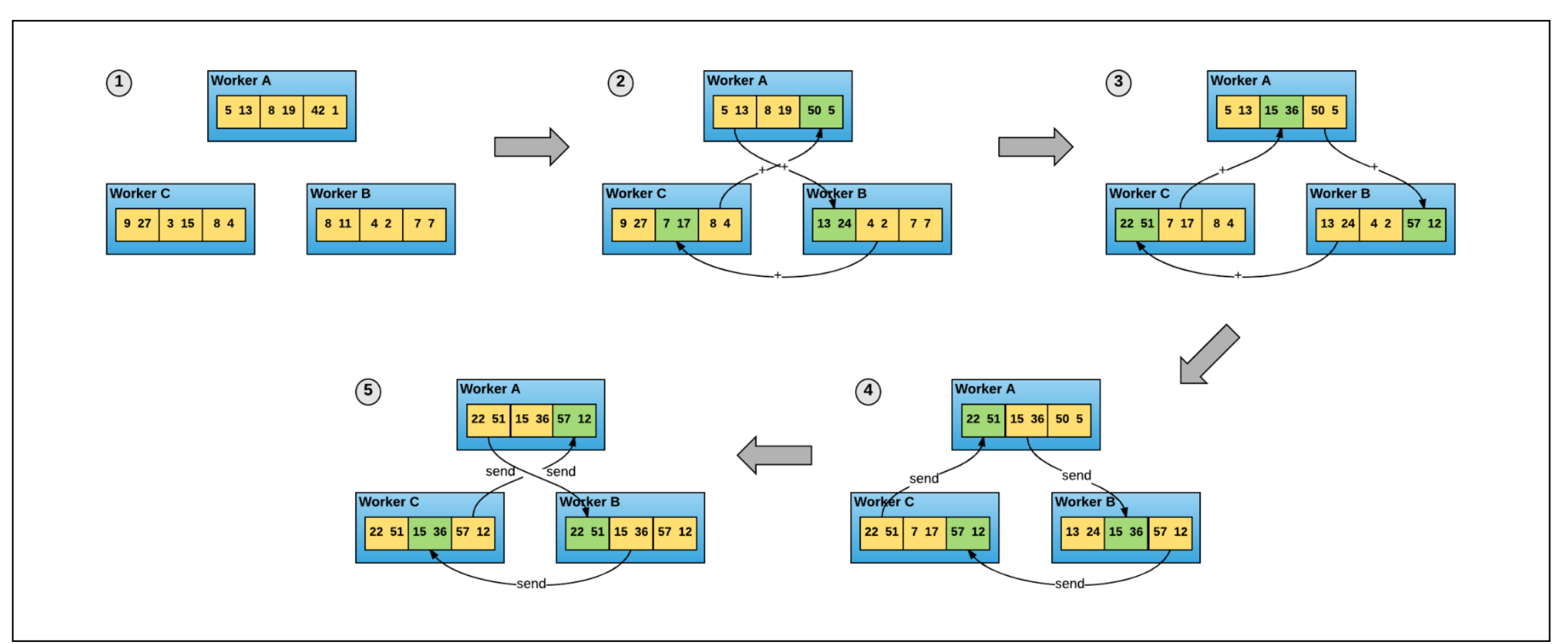Each worker node is connected together to form a closed loop that stores the aggregation of gradient values from all nodes on the worker node by the following algorithm: (-> stands for passing the values to the next worker node, k stands for the array number).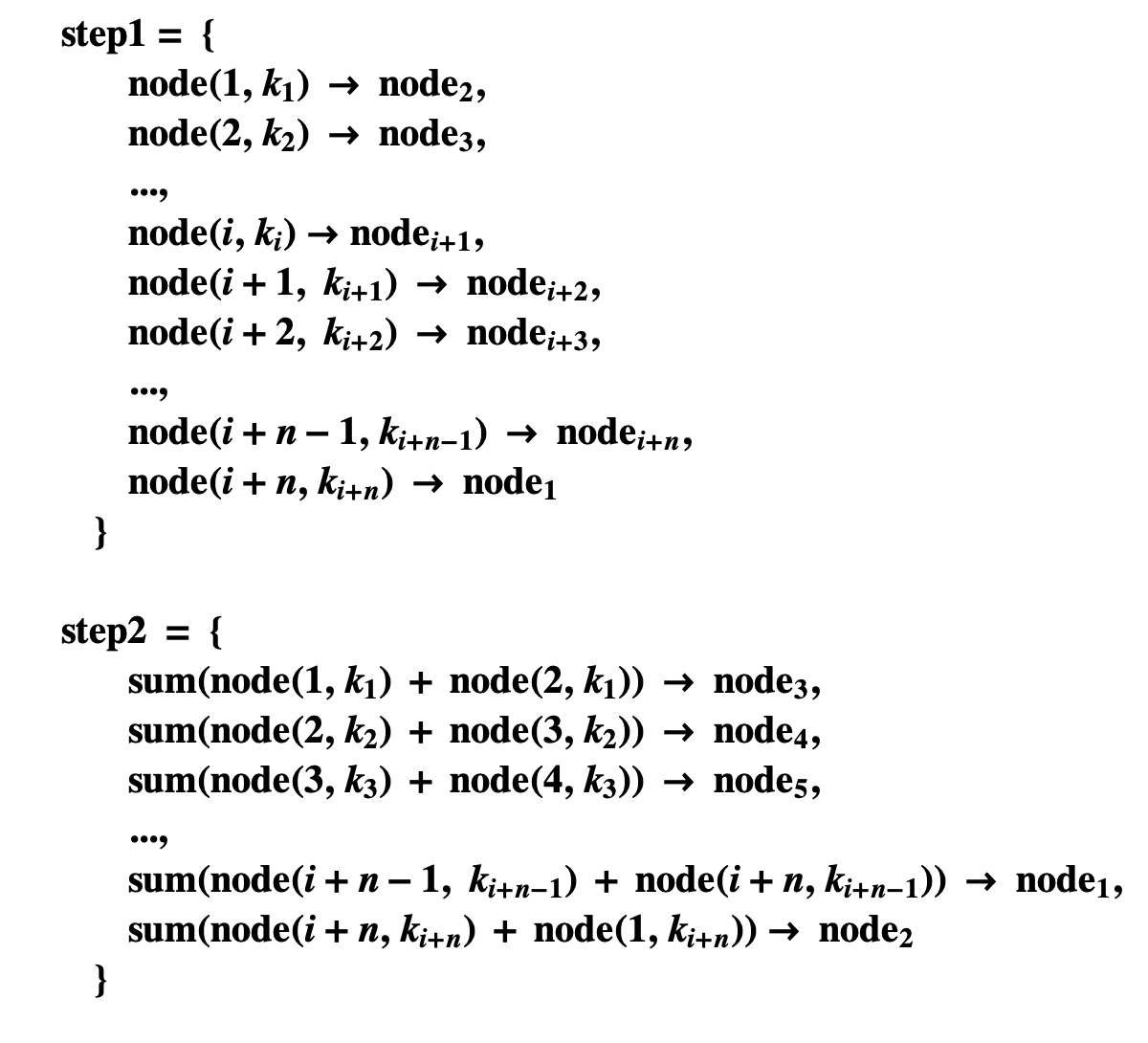The AllReduce feature has been implemented in the MPI message interface, MPICH MPI_Allreduce, and the program is about the ladder. The calculation of the mean value of the gradient requires only an AllReduce() operation, and then the resulting new gradient is passed back to each Worker.

Using horovod requires only the addition of horovod initialization and MPI-based optimizer updates to the program.

The performance utilization comparison between the previous version of TF and the previous version of TF using ring-allreduce: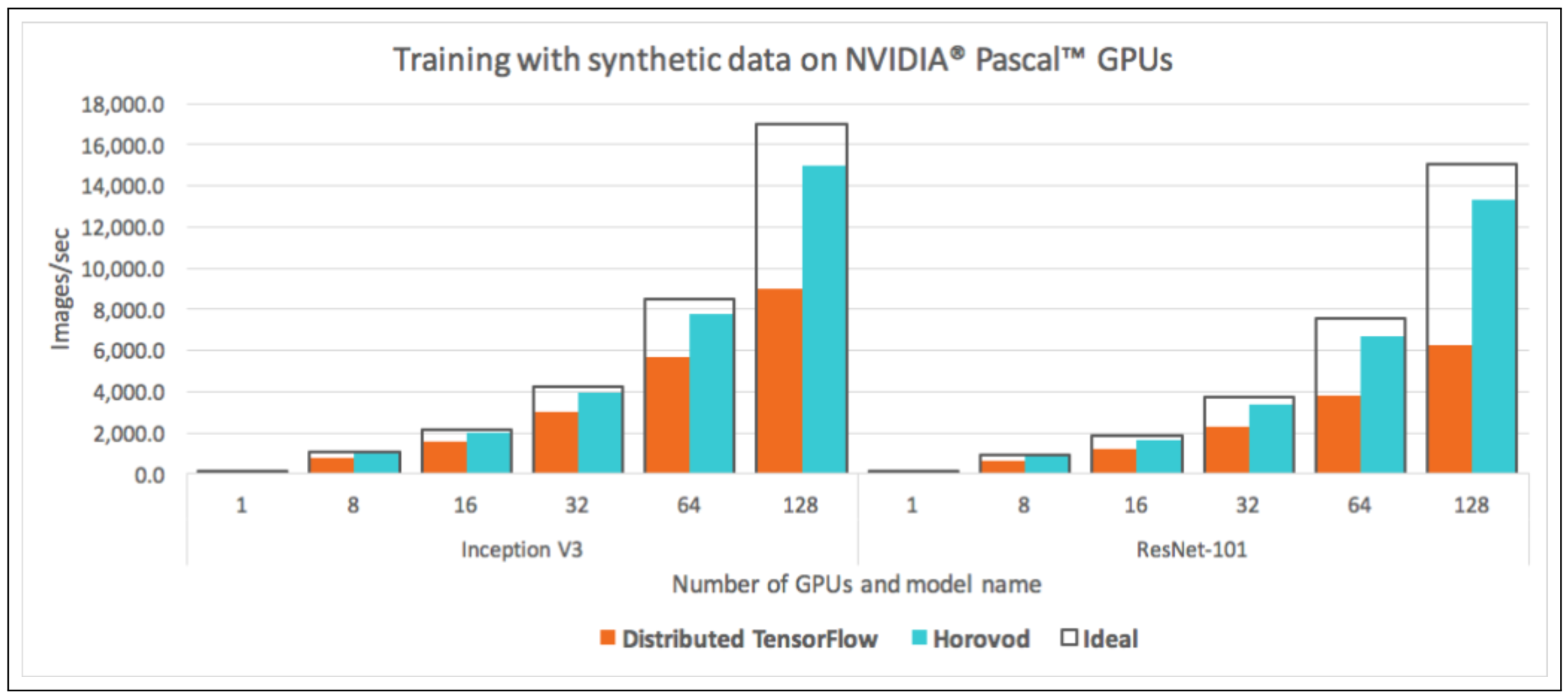All of the following distributed training can be done on a Raspberry Pie 4 cluster.

The code for training MNIST LeNet is as follows:

``````#!/bin/env python3

# -*- coding: utf-8 -*-
"""
Spyder Editor

This is a temporary script file.
"""

import numpy as np
import tensorflow as tf
from tensorflow import keras
import horovod.tensorflow.keras as hvd

hvd.init() // horovod 初始化

mnist = keras.datasets.mnist

(x_train, y_train), (x_test, y_test) = mnist.load_data()

x_train, x_test = x_train / 255.0, x_test / 255.0

x_train,x_test = np.expand_dims(x_train, axis=3), np.expand_dims(x_test, axis=3)

model = keras.models.Sequential([
keras.layers.MaxPool2D(2),
keras.layers.MaxPool2D((2)),
keras.layers.Flatten(),
keras.layers.Dense(512, activation='relu'),
keras.layers.Dense(10, activation='softmax')])

opt = hvd.DistributedOptimizer(opt) // 增加分布式的优化器

model.compile(optimizer=opt,loss='sparse_categorical_crossentropy',
metrics=['accuracy'])

callbacks = [
]

if hvd.rank() == 0:
callbacks.append(keras.callbacks.ModelCheckpoint('./checkpoint-{epoch}.h5'))

model.fit(x_train, y_train,steps_per_epoch=500,
callbacks=callbacks,
epochs=20,
verbose=1 if hvd.rank() == 0 else 0)

``````

## MPICH Version

Build Docker Image Dockerfile

``````FROM ubuntu:20.04
LABEL VERSION="1.0.0"
LABEL maintainer="[email protected]"
RUN apt-get -y update && apt-get -y upgrade
RUN apt-get install -y g++ gcc gfortran openssh-server build-essential make cmake pkg-config mpich libmpich-dev
RUN apt-get install libffi-dev libffi7 libblas-dev liblapack-dev libatlas-base-dev python3-numpy libhdf5-dev -y
RUN apt-get -y -q autoremove && apt-get -y -q clean
RUN sed -i 's/#Port 22/Port 2222/' /etc/ssh/sshd_config

WORKDIR /
RUN mkdir /run/sshd -pv && mkdir /root/.ssh

RUN useradd  -m -s /bin/bash -u 1001 chenfeng
RUN mkdir -pv /home/chenfeng/.ssh
COPY authorized_keys id_rsa.pub id_rsa /root/.ssh/
COPY authorized_keys id_rsa.pub id_rsa /home/chenfeng/.ssh/
RUN chown chenfeng:chenfeng /home/chenfeng/.ssh/authorized_keys
RUN chmod 0600 /root/.ssh/id_rsa && chmod 0600 /home/chenfeng/.ssh/id_rsa
COPY ./tensorflow-2.2.0-cp38-cp38-linux_aarch64.whl /tmp/
RUN pip3 install grpcio
RUN pip3 install scipy=="1.4.1"
RUN pip3 install /tmp/tensorflow-2.2.0-cp38-cp38-linux_aarch64.whl
RUN rm -fv /tmp/tensorflow-2.2.0-cp38-cp38-linux_aarch64.whl
RUN HOROVOD_WITH_TENSORFLOW=1 pip3 install horovod[tensorflow]
EXPOSE 2222
CMD ["/usr/sbin/sshd", "-D"]
``````

## OpenMPI Version

Build DockerImage Dockerfile

``````FROM ubuntu:20.04
LABEL VERSION="1.0.0"
LABEL maintainer="[email protected]"
RUN apt-get -y update && apt-get -y upgrade
RUN apt-get install -y g++ gcc gfortran openssh-server build-essential make cmake pkg-config
RUN apt-get install libffi-dev libffi7 libblas-dev liblapack-dev libatlas-base-dev python3-numpy libhdf5-dev libopenmpi-dev openmpi-bin -y
RUN apt-get -y -q autoremove && apt-get -y -q clean
RUN sed -i 's/#Port 22/Port 2222/' /etc/ssh/sshd_config

WORKDIR /
RUN mkdir /run/sshd -pv && mkdir /root/.ssh
COPY ./ssh_config /etc/ssh/ssh_config

RUN useradd  -m -s /bin/bash -u 1001 chenfeng
RUN mkdir -pv /home/chenfeng/.ssh
COPY authorized_keys id_rsa.pub id_rsa /root/.ssh/
COPY authorized_keys id_rsa.pub id_rsa /home/chenfeng/.ssh/
RUN chown chenfeng:chenfeng /home/chenfeng/.ssh/authorized_keys
RUN chmod 0600 /root/.ssh/id_rsa && chmod 0600 /home/chenfeng/.ssh/id_rsa
COPY ./tensorflow-2.2.0-cp38-cp38-linux_aarch64.whl /tmp/
RUN pip3 install grpcio
RUN pip3 install scipy=="1.4.1"
RUN pip3 install /tmp/tensorflow-2.2.0-cp38-cp38-linux_aarch64.whl
RUN rm -fv /tmp/tensorflow-2.2.0-cp38-cp38-linux_aarch64.whl
RUN HOROVOD_WITH_TENSORFLOW=1 pip3 install horovod[tensorflow]
RUN pip3 install ipython
RUN rm -fv /'=1.8.6'
EXPOSE 2222
CMD ["/usr/sbin/sshd", "-D"]
``````

## Distributed training program testing

Training with 3 * Raspberry pi 4 4G.

Program Code:

cat_vs_dog cnn-distributed.py:

``````#!/usr/bin/env python3
# -*- coding: utf-8 -*-
"""
Created on Fri Jun  5 14:23:53 2020

@author: alexchen
"""

import tensorflow as tf
from tensorflow import keras
from tensorflow.keras.models import Sequential
from tensorflow.keras.layers import Conv2D
from tensorflow.keras.layers import MaxPooling2D
from tensorflow.keras.layers import Flatten
from tensorflow.keras.layers import Dense
import tensorflow_datasets as tfds
import json
import os

import horovod.tensorflow.keras as hvd

hvd.init()

opt = hvd.DistributedOptimizer(opt)

def create_model():
model = Sequential([
Conv2D(32,(3,3),input_shape=(64,64,3),activation='relu'),
MaxPooling2D(pool_size=(2,2)),
Conv2D(32,(3,3),activation='relu'),
MaxPooling2D(pool_size=(2,2)),
Flatten(),
Dense(units=128,activation='relu'),
Dense(units=1, activation='sigmoid')
])
model.compile(optimizer=opt,loss='binary_crossentropy',metrics = ['accuracy'])
return model

split=tfds.Split.TRAIN,
as_supervised=True,
data_dir="./tensorflow_datasets")

def resize(image, label):
image = tf.image.resize(image, [64, 64]) / 255.0
return image, label

callbacks = [
]

if hvd.rank() == 0:
callbacks.append(keras.callbacks.ModelCheckpoint('./checkpoint-{epoch}.h5'))

batch_size = 32
multi_worker_dataset = dataset.repeat().map(resize).shuffle(1024).batch(batch_size)
model = create_model()

model.fit(multi_worker_dataset,
callbacks=callbacks,
steps_per_epoch = 50,
epochs = 50,
verbose=1 if hvd.rank() == 0 else 0)
``````
``````horovodrun --verbose -np 3 --network-interface eth0  --hostfile ./hosts -p 2222 python3 cnn-distributed.py
``````

Result: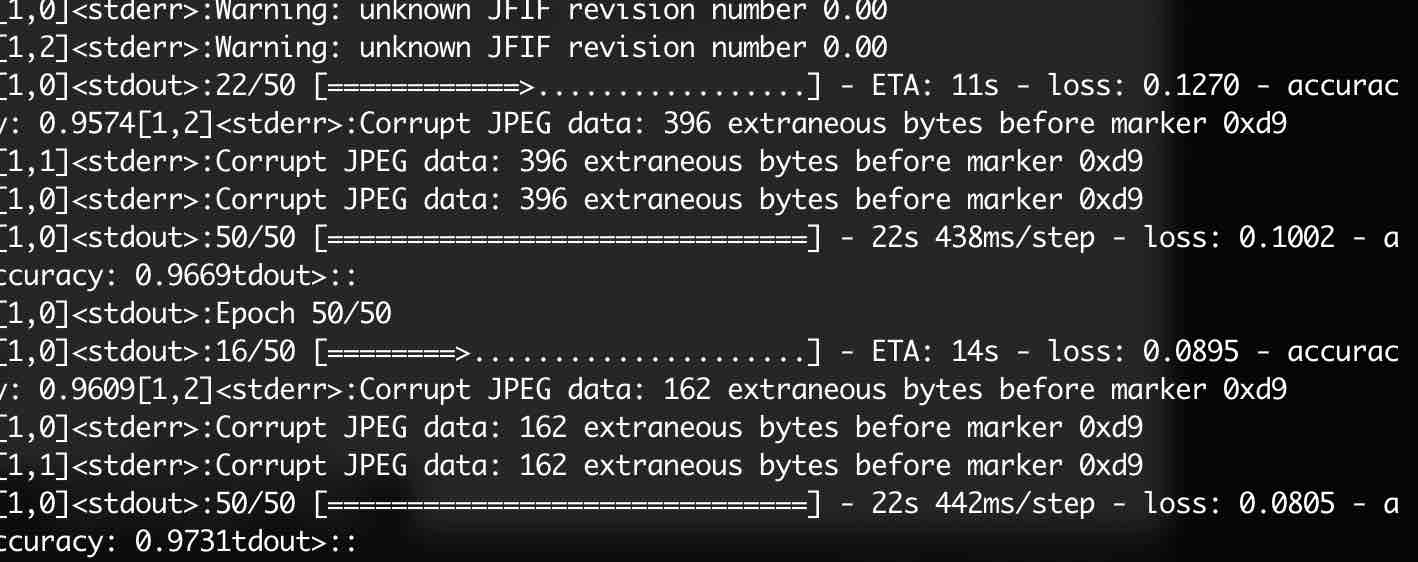If we use a single-stage training model:

``````horovodrun --verbose -np 1  python3 cnn-distributed.py
``````

Result: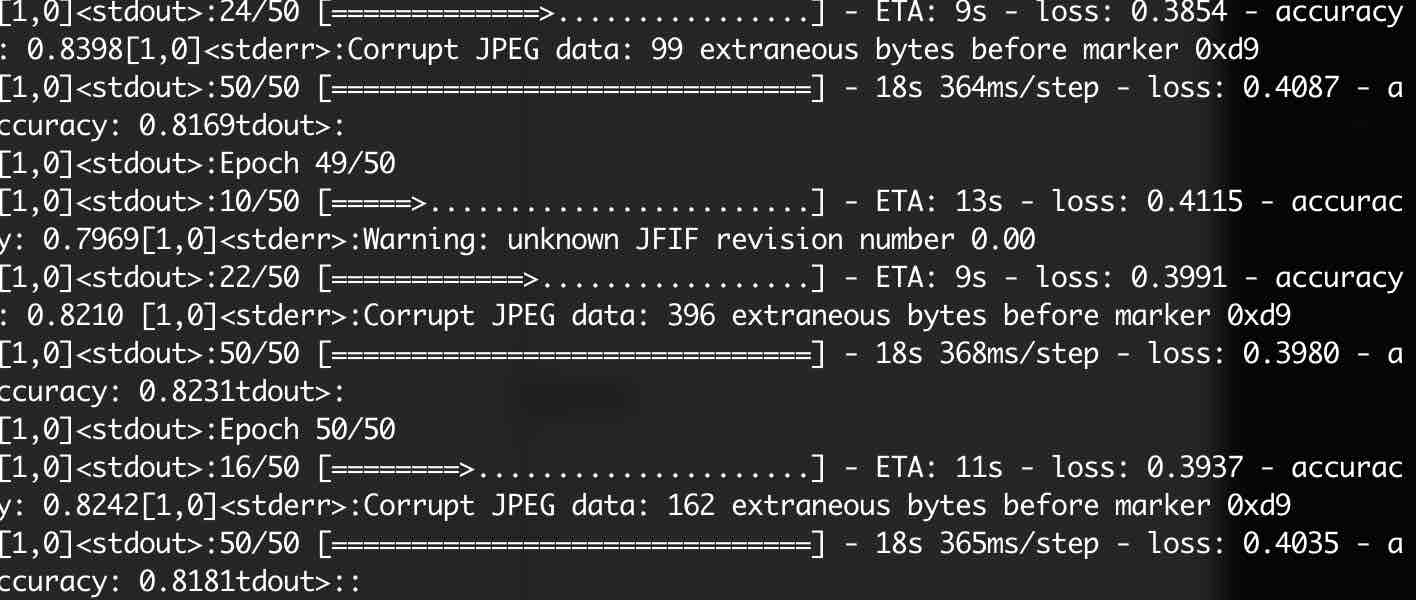The accuracy of the model converges faster when using distributed training, and the throughput of training data is higher when training on multiple machines than when training on a single machine.